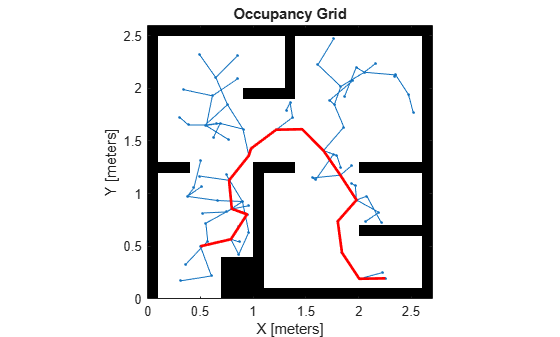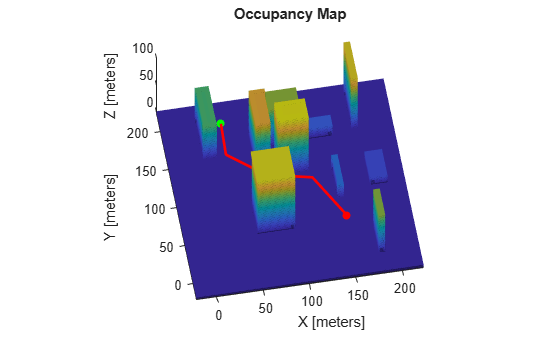# plannerRRT

Create an RRT planner for geometric planning

Since R2019b

## Description

The `plannerRRT` object creates a rapidly-exploring random tree (RRT) planner for solving geometric planning problems. RRT is a tree-based motion planner that builds a search tree incrementally from samples randomly drawn from a given state space. The tree eventually spans the search space and connects the start state to the goal state. The general tree growing process is as follows:

1. The planner samples a random state xrand in the state space.

2. The planner finds a state xnear that is already in the search tree and is closest (based on the distance definition in the state space) to xrand.

3. The planner expands from xnear towards xrand, until a state xnew is reached.

4. Then new state xnew is added to the search tree.

For geometric RRT, the expansion and connection between two states can be found analytically without violating the constraints specified in the state space of the planner object.

## Creation

### Syntax

``planner = plannerRRT(stateSpace,stateVal)``
``planner = plannerRRT(___,Name=Value)``

### Description

````planner = plannerRRT(stateSpace,stateVal)` creates an RRT planner from a state space object, `stateSpace`, and a state validator object, `stateVal`. The state space of `stateVal` must be the same as `stateSpace`. `stateSpace` and `stateVal` also sets the StateSpace and StateValidator properties of the `planner`. ```

example

````planner = plannerRRT(___,Name=Value)` sets properties using one or more name-value arguments in addition to the input arguments in the previous syntax. You can specify the StateSampler, MaxNumTreeNodes, MaxIterations, MaxConnectionDistance, GoalReachedFcn, and GoalBias properties as name-value arguments.```

## Properties

expand all

State space for the planner, specified as a state space object. You can use state space objects such as `stateSpaceSE2`, `stateSpaceDubins`, `stateSpaceReedsShepp`, and `stateSpaceSE3`. You can also customize a state space object using the `nav.StateSpace` object.

State validator for the planner, specified as a state validator object. You can use state validator objects such as `validatorOccupancyMap`, `validatorVehicleCostmap`, and `validatorOccupancyMap3D`.

Since R2023b

State space sampler used for finding state samples in the input space, specified as a `stateSamplerUniform` object, `stateSamplerGaussian` object, `stateSamplerMPNET` object, or `nav.StateSampler` object. By default, the `plannerRRT` uses uniform state sampling.

Maximum number of nodes in the search tree (excluding the root node), specified as a positive integer.

Example: `MaxNumTreeNodes=2500`

Data Types: `single` | `double`

Maximum number of iterations, specified as a positive integer.

Example: `MaxIterations=2500`

Data Types: `single` | `double`

Maximum length of a motion allowed in the tree, specified as a scalar.

Example: `MaxConnectionDistance=0.3`

Data Types: `single` | `double`

Callback function to evaluate whether the goal is reached, specified as a function handle. You can create your own goal reached function. The function must follow this syntax:

` function isReached = myGoalReachedFcn(planner,currentState,goalState)`

where:

• `planner` — The created planner object, specified as `plannerRRT` object.

• `currentState` — The current state, specified as a three element real vector.

• `goalState` — The goal state, specified as a three element real vector.

• `isReached` — A boolean variable to indicate whether the current state has reached the goal state, returned as `true` or `false`.

To use custom `GoalReachedFcn` in code generation workflow, this property must be set to a custom function handle before calling the plan function and it cannot be changed after initialization.

Data Types: `function handle`

Probability of choosing the goal state during state sampling, specified as a real scalar in range [0,1]. The property defines the probability of choosing the actual goal state during the process of randomly selecting states from the state space. You can start by setting the probability to a small value such as `0.05`.

Example: `GoalBias=0.1`

Data Types: `single` | `double`

## Object Functions

 `plan` Plan path between two states `copy` Create copy of planner object

## Examples

collapse all

Create a state space.

`ss = stateSpaceSE2;`

Create an `occupancyMap`-based state validator using the created state space.

`sv = validatorOccupancyMap(ss);`

Create an occupancy map from an example map and set map resolution as 10 cells/meter.

```load exampleMaps map = occupancyMap(simpleMap,10); sv.Map = map;```

Set validation distance for the validator.

`sv.ValidationDistance = 0.01;`

Update state space bounds to be the same as map limits.

`ss.StateBounds = [map.XWorldLimits;map.YWorldLimits;[-pi pi]];`

Create the path planner and increase the maximum connection distance.

`planner = plannerRRT(ss,sv,MaxConnectionDistance=0.3);`

Set the start and goal states.

```start = [0.5 0.5 0]; goal = [2.5 0.2 0];```

Plan a path with default settings.

```rng(100,'twister'); % for repeatable result [pthObj,solnInfo] = plan(planner,start,goal);```

Visualize the results.

```show(map) hold on % Tree expansion plot(solnInfo.TreeData(:,1),solnInfo.TreeData(:,2),'.-') % Draw path plot(pthObj.States(:,1),pthObj.States(:,2),'r-','LineWidth',2)```Load a 3-D occupancy map of a city block into the workspace. Specify the threshold to consider cells as obstacle-free.

```mapData = load("dMapCityBlock.mat"); omap = mapData.omap; omap.FreeThreshold = 0.5;```

Inflate the occupancy map to add a buffer zone for safe operation around the obstacles.

`inflate(omap,1)`

Create an SE(3) state space object with bounds for state variables.

`ss = stateSpaceSE3([0 220;0 220;0 100;inf inf;inf inf;inf inf;inf inf]);`

Create a 3-D occupancy map state validator using the created state space. Assign the occupancy map to the state validator object. Specify the sampling distance interval.

```sv = validatorOccupancyMap3D(ss, ... Map = omap, ... ValidationDistance = 0.1);```

Create a RRT path planner with increased maximum connection distance and reduced maximum number of iterations. Specify a custom goal function that determines that a path reaches the goal if the Euclidean distance to the target is below a threshold of 1 meter.

```planner = plannerRRT(ss,sv, ... MaxConnectionDistance = 50, ... MaxIterations = 1000, ... GoalReachedFcn = @(~,s,g)(norm(s(1:3)-g(1:3))<1), ... GoalBias = 0.1);```

Specify start and goal poses.

```start = [40 180 25 0.7 0.2 0 0.1]; goal = [150 33 35 0.3 0 0.1 0.6];```

Configure the random number generator for repeatable result.

`rng(1,"twister");`

Plan the path.

`[pthObj,solnInfo] = plan(planner,start,goal);`

Visualize the planned path.

```show(omap) axis equal view([-10 55]) hold on % Start state scatter3(start(1,1),start(1,2),start(1,3),"g","filled") % Goal state scatter3(goal(1,1),goal(1,2),goal(1,3),"r","filled") % Path plot3(pthObj.States(:,1),pthObj.States(:,2),pthObj.States(:,3), ... "r-",LineWidth=2)```S.M. Lavalle and J.J. Kuffner. "Randomized Kinodynamic Planning." The International Journal of Robotics Research. Vol. 20, Number 5, 2001, pp. 378 – 400.